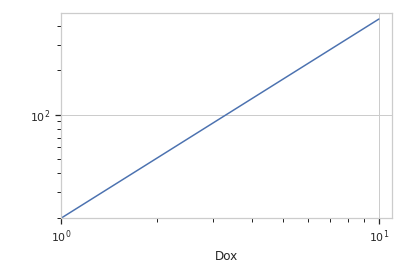# cytoflow.views.stats_1d¶

class cytoflow.views.stats_1d.Stats1DView[source]

Plot a statistic. The value of the statistic will be plotted on the Y axis; a numeric conditioning variable must be chosen for the X axis. Every variable in the statistic must be specified as either the variable or one of the plot facets.

variable_scale

The scale applied to the variable (on the X axis)

Type: {‘linear’, ‘log’, ‘logicle’}
statistic

The name of the statistic to plot. Must be a key in the statistics attribute of the Experiment being plotted.

Type: (str, str)
error_statistic

The name of the statistic used to plot error bars. Must be a key in the statistics attribute of the Experiment being plotted.

Type: (str, str)
scale

The scale applied to the data before plotting it.

Type: {‘linear’, ‘log’, ‘logicle’}
variable

The condition that varies when plotting this statistic: used for the x axis of line plots, the bar groups in bar plots, etc.

Type: str
subset

An expression that specifies the subset of the statistic to plot.

Type: str
xfacet, yfacet

Set to one of the conditions in the Experiment, and a new row or column of subplots will be added for every unique value of that condition.

Type: String
huefacet

Set to one of the conditions in the in the Experiment, and a new color will be added to the plot for every unique value of that condition.

Type: String
huescale

How should the color scale for huefacet be scaled?

Type: {‘linear’, ‘log’, ‘logicle’}

Examples

Make a little data set.

>>> import cytoflow as flow
>>> import_op = flow.ImportOp()
>>> import_op.tubes = [flow.Tube(file = "Plate01/RFP_Well_A3.fcs",
...                              conditions = {'Dox' : 10.0}),
...                    flow.Tube(file = "Plate01/CFP_Well_A4.fcs",
...                              conditions = {'Dox' : 1.0})]
>>> import_op.conditions = {'Dox' : 'float'}
>>> ex = import_op.apply()


Create and a new statistic.

>>> ch_op = flow.ChannelStatisticOp(name = 'MeanByDox',
...                     channel = 'Y2-A',
...                     function = flow.geom_mean,
...                     by = ['Dox'])
>>> ex2 = ch_op.apply(ex)


View the new statistic

>>> flow.Stats1DView(variable = 'Dox',
...                  statistic = ('MeanByDox', 'geom_mean'),
...                  variable_scale = 'log',
...                  scale = 'log').plot(ex2)enum_plots(experiment)[source]

Returns an iterator over the possible plots that this View can produce. The values returned can be passed to plot().

plot(experiment, plot_name=None, **kwargs)[source]

Plot a chart of a variable’s values against a statistic.

Parameters: experiment (Experiment) – The Experiment to plot using this view. title (str) – Set the plot title xlabel, ylabel (str) – Set the X and Y axis labels huelabel (str) – Set the label for the hue facet (in the legend) legend (bool) – Plot a legend for the color or hue facet? Defaults to True. sharex, sharey (bool) – If there are multiple subplots, should they share axes? Defaults to True. row_order, col_order, hue_order (list) – Override the row/column/hue facet value order with the given list. If a value is not given in the ordering, it is not plotted. Defaults to a “natural ordering” of all the values. height (float) – The height of each row in inches. Default = 3.0 aspect (float) – The aspect ratio of each subplot. Default = 1.5 col_wrap (int) – If xfacet is set and yfacet is not set, you can “wrap” the subplots around so that they form a multi-row grid by setting col_wrap to the number of columns you want. sns_style ({“darkgrid”, “whitegrid”, “dark”, “white”, “ticks”}) – Which seaborn style to apply to the plot? Default is whitegrid. sns_context ({“paper”, “notebook”, “talk”, “poster”}) – Which seaborn context to use? Controls the scaling of plot elements such as tick labels and the legend. Default is talk. despine (Bool) – Remove the top and right axes from the plot? Default is True. orientation ({‘vertical’, ‘horizontal’}) lim ((float, float)) – Set the range of the plot’s axis. variable_lim ((float, float)) – The limits on the variable axis color (a matplotlib color) – The color to plot with. Overridden if huefacet is not None linewidth (float) – The width of the line, in points linestyle ([‘solid’ | ‘dashed’, ‘dashdot’, ‘dotted’ | (offset, on-off-dash-seq) | ‘-’ | ‘–’ | ‘-.’ | ‘:’ | ‘None’ | ‘ ‘ | ‘’]) marker (a matplotlib marker style) – See http://matplotlib.org/api/markers_api.html#module-matplotlib.markers markersize (int) – The marker size in points markerfacecolor (a matplotlib color) – The color to make the markers. Overridden (?) if huefacet is not None alpha (the alpha blending value, from 0.0 (transparent) to 1.0 (opaque)) capsize (scalar) – The size of the error bar caps, in points shade_error (bool) – If False (the default), plot the error statistic as traditional “error bars.” If True, plot error statistic as a filled, shaded region. shade_alpha (float) – The transparency of the shaded error region, from 0.0 (transparent) to 1.0 (opaque.) Default is 0.2.

Notes

Other kwargs are passed to matplotlib.pyplot.plot